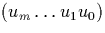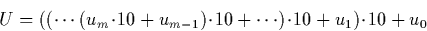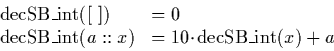Next: Decimal fractions to signed Up: Decimal to Signed Binary Previous: Decimal to Signed Binary

### Decimal integers to signed binary

Conversion from decimal (base 10) can be performed using binary (base 2) arithmetic as follows: Suppose we have the decimal integer. We compute the binary representation U asThis can be easily computed using the signed binary (mantissa, exponent) operations which we develop algorithms for in chapter 4. We extract the finite decimal integer, reverse the digits, and present them to a recursive algorithm which has a base case which returns zero when the input is exhausted, but otherwise computes number represented by the tail of the list, multiplies this by ten, and adds the number at the head of the list:Martin Escardo
5/11/2000